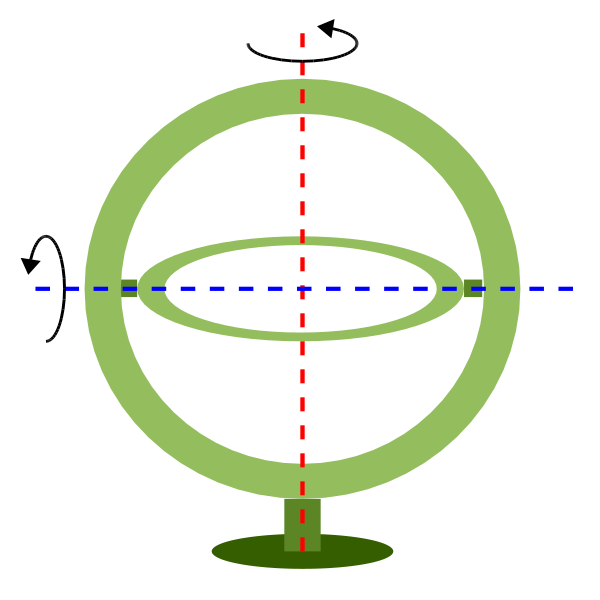# Geostationary Satellite View¶

The geos projection pictures how a geostationary satellite scans the earth at regular scanning angle intervals.

 Classification Azimuthal Available forms Forward and inverse, spherical and elliptical projection Defined area Global Alias geos Domain 2D Input type Geodetic coordinates Output type Projected coordinatesproj-string: +proj=geos +h=35785831.0 +lon_0=-60 +sweep=y

## Usage¶

In order to project using the geos projection you can do the following:

proj +proj=geos +h=35785831.0


The required argument h is the viewing point (satellite position) height above the earth.

The projection coordinate relate to the scanning angle by the following simple relation:

scanning_angle (radians) = projection_coordinate / h


### Note on sweep angle¶

The viewing instrument on-board geostationary satellites described by this projection have a two-axis gimbal viewing geometry. This means that the different scanning positions are obtained by rotating the gimbal along a N/S axis (or y) and a E/W axis (or x).In the image above, the outer-gimbal axis, or sweep-angle axis, is the N/S axis (y) while the inner-gimbal axis, or fixed-angle axis, is the E/W axis (x).

This example represents the scanning geometry of the Meteosat series satellite. However, the GOES satellite series use the opposite scanning geometry, with the E/W axis (x) as the sweep-angle axis, and the N/S (y) as the fixed-angle axis.

The sweep argument is used to tell PROJ which on which axis the outer-gimbal is rotating. The possible values are x or y, y being the default. Thus, the scanning geometry of the Meteosat series satellite should take sweep as y, and GOES should take sweep as x.

## Parameters¶

### Required¶

+h=<value>

Height of the view point above the Earth and must be in the same units as the radius of the sphere or semimajor axis of the ellipsoid.

### Optional¶

+sweep=<axis>

Sweep angle axis of the viewing instrument. Valid options are “x” and “y”.

Defaults to “y”.

+lon_0=<value>

Longitude of projection center.

Defaults to 0.0.

+R=<value>

Radius of the sphere given in meters. If used in conjunction with +ellps +R takes precedence.

+ellps=<value>

See proj -le for a list of available ellipsoids.

Defaults to “GRS80”.

+x_0=<value>

False easting.

Defaults to 0.0.

+y_0=<value>

False northing.

Defaults to 0.0.